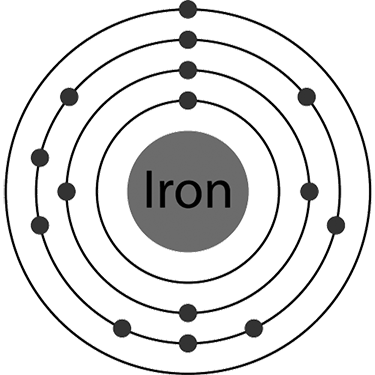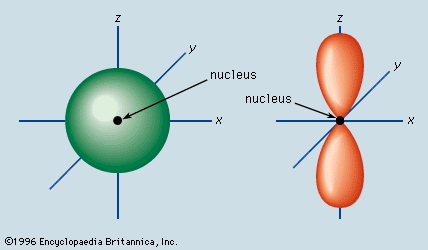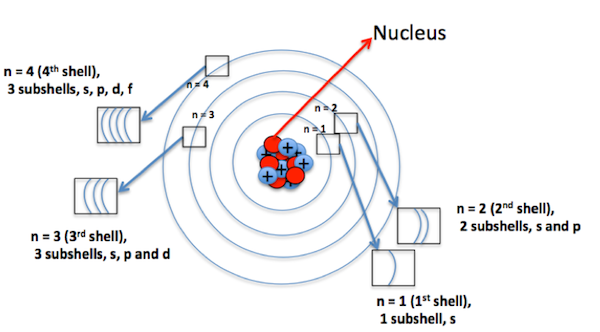# Quantum Numbers Study Guide

INTRODUCTION

Imagine 56 electrons in iron moving simultaneously around just one nucleus. Don’t you think the actual picture will be chaotic? It sounds chaotic, but interestingly, it isn’t.Source

Every electron in an atom has some fixed numbers, which tell us about its location. So, something that we thought to be unorganized and chaotic is beautiful. Let’s explore what these numbers are and how they help us find the location of an electron!

### THE NUMBERS

The numbers which specify the location of each electron are called Quantum Numbers. There are four quantum numbers in total. Let’s understand them in a bit more detail!

### PRINCIPAL QUANTUM NUMBER(n):

An atom is arranged in shells that surround the nucleus. Shells are named K, L, M, N, and so on. The shell closest to the nucleus is K, given the principal quantum number n =1. The distance between the nucleus and electrons keeps increasing for the subsequent shells or energy levels; n can have values 1, 2, 3, 4, etc.

### ANGULAR QUANTUM NUMBER(ℓ):

It is the quantum number that tells about the angular momentum of an electron. It tells about the shape of the orbital in which the electron resides. For example, an electron in s orbital moves in a sphere as the shape of s orbital is spherical.Source

The value of ℓ is dependent on the principal quantum number(n) as ℓ =0 to n -1, but it is always positive. For n=3, the possible values are 0 to n-1 that are 0,1,2.

### MAGNETIC QUANTUM NUMBER(mℓ):

It tells about the orientation of the orbital in space. mℓ can have values -ℓ to ℓ. So, in total, the values are 2ℓ 1.For ℓ = 1, mℓ can have values -1 to 1. That are -1,0, 1. In total, three values.Source

### SPIN QUANTUM NUMBER(ms):

This quantum number tells about the spin of an electron. There are only these two possibilities of movement of an electron; ms has the values of -½ or ½. The spins can also be represented as ‘spin up ( ↑)’ and ‘spin down( ↓). A single orbital can accommodate 2 electrons with opposite spins.

Do you know what the best part of quantum numbers is?

No 2 electrons can have all four same quantum numbers. Like your neighbor, who will share almost the same address except for the house number.

## CONCLUSION

• The numbers which specify the location of each electron are called Quantum Numbers.
• The four quantum numbers are principal, angular momentum, and magnetic and spin quantum numbers.

### FAQs:

1. What are quantum numbers?

The numbers which specify the location of each electron are called Quantum Numbers.

2. What are the possible ℓ values for n=2?

The possible values are 0 and 1 as ℓ can have values from 0 to n-1.

3. What are the possible values of ms?

It can have two values as -½ or ½.

We hope you enjoyed studying this lesson and learned something cool about Quantum Numbers! Join our Discord community to get any questions you may have answered and engage with other students like you! Don’t forget to download our App to experience our fun VR classrooms – we promise it makes studying much more fun! 😎

## SOURCES

1. Quantum Numbers: https://www.ck12.org/c/chemistry/quantum-numbers/lesson/Quantum-Numbers-CHEM/?referrer=concept_details. Accessed 7th March 2022.
2. Angular Momentum Quantum Number: https://study.com/academy/lesson/angular-momentum-quantum-number-definition-example-quiz.html. Accessed 7th March 2022.
]]>